# Samacheer Kalvi Books: Tamilnadu State Board Text Books Solutions

## Samacheer Kalvi 10th Maths Chapter 1 Relations and Functions Notes PDF Download: Tamil Nadu STD 10th Maths Chapter 1 Relations and Functions NotesSamacheer Kalvi 10th Maths Chapter 1 Relations and Functions Notes PDF Download: Tamil Nadu STD 10th Maths Chapter 1 Relations and Functions Notes

## Samacheer Kalvi 10th Maths Chapter 1 Relations and Functions Notes PDF Download

We bring to you specially curated Samacheer Kalvi 10th Maths Chapter 1 Relations and Functions Notes PDF which have been prepared by our subject experts after carefully following the trend of the exam in the last few years. The notes will not only serve for revision purposes, but also will have several cuts and easy methods to go about a difficult problem.

 Board Tamilnadu Board Study Material Notes Class Samacheer Kalvi 10th Maths Subject 10th Maths Chapter Chapter 1 Relations and Functions Format PDF Provider Samacheer Kalvi Books

## How to Download Samacheer Kalvi 10th Maths Chapter 1 Relations and Functions Notes PDFs?

2. Click on the Samacheer Kalvi 10th Maths Notes PDF.
3. Look for your preferred subject.
4. Now download the Samacheer Kalvi 10th Maths Chapter 1 Relations and Functions notes PDF.

## Download Samacheer Kalvi 10th Maths Chapter 1 Relations and Functions Chapterwise Notes PDF

10th Maths Exercise 1.1 Samacheer Kalvi Question 1.
Find A × B, A × A and B × A
(i) A = {2,-2,3} and B = {1,-4}
(ii) A = B = {p,q]
(iii) A= {m,n} ; B = (Φ)
Solution:
(i) A = {2,-2,3}, B = {1,-4}
A × B = {(2, 1), (2, -4), (-2, 1), (-2, -4), (3,1) , (3,-4)}
A × A = {(2, 2), (2,-2), (2, 3), (-2, 2), (-2, -2), (-2, 3), (3, 2), (3, -2), (3,3) }
B × A = {(1, 2), (1, -2), (1, 3), (-4, 2), (-4, -2), (-4,3)}

(ii) A = B = {(p,q)]
A × B = {(p, p), {p, q), (q, p), (q, q)}
A × A = {(p, p), (p, q), (q, p), (q, q)}
B × A = {(p,p), {p, q), (q, p), (q, q)}

(iii) A = {m,n} × Φ
A × B = { }
A × A = {(m, m), (m, n), (n, m), (n, n)}
B × A = { }

10th Maths Exercise 1.1 Question 2.
Let A= {1,2,3} and B = {× | x is a prime number less than 10}. Find A × B and B × A.
A = {1,2,3}, B = {2, 3, 5, 7}
A × B = {1,2,3} × {2, 3, 5, 7}
= {(1, 2) (1, 3) (1, 5) (1, 7) (2, 2)
(2, 3) (2, 5) (2, 7)(3, 2) (3, 3) (3, 5) (3, 7)}
B × A = {2, 3, 5, 7} × {1,2,3}
= {(2, 1)(2, 2)(2, 3)(3, 1)(3, 2)(3, 3) (5, 1)(5, 2)(5, 3) (7, 1) (7,2)(7, 3)}

10th Maths Exercise 1.1 In Tamil Question 3.
If B × A = {(-2, 3),(-2, 4),(0, 3),(0, 4),(3, 3), (3, 4)} find A and B.
Solution:
B × A ={(-2, 3), (-2, 4), (0, 3), (0, 4), (3, 3), (3, 4)}
A = {3, 4), B = { -2, 0, 3}

Samacheer Kalvi 10th Maths Exercise 1.1 Question 4.
If A= {5, 6}, B = {4, 5 ,6}, C = {5, 6, 7}, Show that A × A = (B × B) ∩ (C × C)
A ={5,6}, B = {4,5,6}, C = {5, 6,7}
A × A = {5, 6} × {5,6}
= {(5, 5) (5, 6) (6, 5) (6, 6)} ….(1)
B × B = {4, 5, 6} × {4, 5, 6}
= {(4, 4)(4, 5)(4, 6)(5, 4)(5, 5) (5, 6) (6, 4)(6, 5) (6, 6)}
C × C = {5,6,7} × {5,6,7}
= {(5, 5)(5, 6)(5, 7)(6, 5)(6, 6) (6, 7)(7, 5)(7, 6) (7, 7)}
(B × B) ∩ (C × C) = {(5, 5)(5, 6)(6, 5)(6, 6)} ….(2)
From (1) and (2) we get
A × A = (B × B) ∩ (C × C)

Ex 1.1 Class 10 Samacheer Question 5.
Given A ={1, 2, 3}, B = {2, 3, 5}, C = {3, 4} and D = {1, 3, 5}, check if (A ∩ C) x (B ∩ D) = (A × B) ∩ (C × D) is true?
Solution:
LHS = {(A∩C) × (B∩D)
A ∩C = {3}
B ∩D = {3, 5}
(A ∩ C) × (B ∩ D) = {(3, 3) (3, 5)} ………….. (1)
RHS = (A × B) ∩ (C × D)
A × B = {(1, 2), (1, 3), (1, 5), (2, 2), (2, 3), (2, 5), (3, 2), (3, 3), (3, 5)}
C × D = {(3, 1), (3, 3), (3, 5), (4, 1), (4, 3), (4, 5)}
(A × B) ∩ (C × D) = {(3, 3), (3, 5)} …(2)
∴ (1) = (2) ∴ It is true.

10th Maths Book Exercise 1.1 Question 6.
Let A = {x ∈ W | x < 2},
B = {x ∈ N | 1 < 1 < × < 4} and
C = {3,5}. Verify that
(i) A × (B ∪ C) = (A × B) ∪ (A × C)
(ii) A × (B ∩ C) = (A × B) ∩ (A × C)
(iii) (A ∪ B) × C = (A × C) ∪ (B × C)
(i) A = {0, 1}
B = {2,3,4}
C = {3,5}
(i) A × (B ∪ C) = (A × B) ∪ (A × c)
B ∪ C = {2, 3,4} ∪ {3,5}
= {2, 3, 4, 5}
A × (B ∪ C) = {0, 1} × {2, 3, 4, 5}
= {(0, 2) (0, 3) (0, 4) (0, 5) (1, 2) (1, 3)(1, 4)(1, 5)} ….(1)
A × B = {0, 1} × {2,3,4}
= {(0,2) (0,3) (0,4) (1,2) (1,3) (1,4) }
A × C = {0, 1} × {3, 5}
{(0, 3) (0, 5) (1,3) (1,5)}
(A × B) ∪ (A × C) = {(0, 2) (0, 3) (0, 4) (0, 5) (1, 2)(1, 3)(1, 4)(1, 5)} ….(2)
From (1) and (2) we get
A × (B ∪ C) = (A × B) ∪ (A × C)

(ii) A × (B n C) = (A × B) n (A × C)
B ∩ C = {2,3,4} ∩ {3,5}
= {3}
A × (B ∩ C) = {0, 1} × {3}
= {(0,3) (1,3)} ….(1)
A × B = {0,1} × {2,3,4}
= {(0, 2) (0, 3) (0, 4) (1,2) (1,3) (1,4)}
A × C = {0,1} × {3,5}
{(0, 3) (0, 5) (1,3) (1,5)}
(A × B) n (A × C) = {(0, 3) (1, 3)} ….(2)
From (1) and (2) we get
A × ( B n C) = (A × B) n (A × C)

(iii) (A ∪ B) × C = (A × C) ∪ (B × C)
A ∪ B = {0, 1} ∪ {2,3,4}
= {0,1, 2, 3, 4}
(A ∪ B) × C = {0, 1,2, 3,4} × {3,5}
= {(0, 3) (0, 5) (1, 3) (1, 5)(2, 3) (2, 5) (3, 3)(3, 5) (4, 3)(4, 5)} ….(1)
A × C = {0, 1} × {3,5}
= {(0,3) (0,5) (1,3) (1,5)}
B × C = {2,3,4} × {3,5}
= {(2,3) (2,5) (3,3) (3,5)(4,3)(4,5)}
(A × C) ∪ (B × C) = {(0, 3) (0, 5) (1, 3) (1, 5) (2, 3)(2, 5) (3, 3) (3, 5) (4, 3) (4, 5)} ….(2)
From (1) and (2) we get
(A ∪ B) × C = (A × C) ∪ (B × C)

Maths Exercise 1.1 Class 10 Samacheer Question 7.
Let A = The set of all natural numbers less than 8, B = The set of all prime numbers less than 8, C = The set of even prime number. Verify that
(i) (A ∩ B) × c = (A × C) ∩ (B × C)
(ii) A × (B – C ) = (A × B) – (A × C)
A = {1, 2, 3, 4, 5, 6, 7}
B = {2, 3, 5, 7}
C = {2}
Solution:
(i)(A ∩ B) × C = (A × c) ∩ (B × C)
LHS = (A ∩ B) × C
A ∩ B = {2, 3, 5, 7}
(A ∩ B) × C = {(2, 2), (3, 2), (5, 2), (7, 2)} ………… (1)
RHS = (A × C) ∩ (B × C)
(A × C) = {(1, 2), (2, 2), (3, 2), (4, 2), (5, 2), (6, 2), (7, 2)}
(B × C) = {2, 2), (3, 2), (5, 2), (7, 2)}
(A × C) ∩ (B × C) = {(2, 2), (3, 2), (5, 2), (7, 2)} ……….. (2)
(1) = (2)
∴ LHS = RHS. Hence it is verified.

(ii) A × (B – C) = (A × B) – (A × C)
LHS = A × (B – C)
(B – C) = {3,5,7}
A × (B – C) = {(1, 3), (1, 5), (1, 7), (2, 3), (2, 5), (2, 7) , (3, 3), (3, 5), (3, 7), (4, 3), (4, 5), (4, 7), (5, 3), (5, 5), (5, 7), (6, 3) , (6, 5), (6, 7), (7, 3), (7, 5), (7, 7)} …………. (1)
RHS = (A × B) – (A × C)
(A × B) = {(1,2), (1,3), (1,5), (1,7),
(2, 2), (2, 3), (2, 5), (2, 7),
(3, 2), (3, 3), (3, 5), (3, 7),
(4, 2), (4, 3), (4, 5), (4, 7),
(5, 2), (5, 3), (5, 5), (5, 7),
(6, 2), (6, 3), (6, 5), (6, 7),
(7, 2), (7, 3), (7, 5), (7,7)}
(A × C) = {(1, 2), (2, 2),(3, 2),(4, 2), (5, 2), (6, 2), (7, 2)}
(A × B) – (A × C) = {(1, 3), (1, 5), (1, 7), (2, 3), (2, 5), (2, 7), (3, 3), (3, 5), (3, 7), (4, 3), (4, 5), (4, 7), (5, 3), (5, 5), (5, 7), (6, 3), (6, 5), (6, 7), (7, 3), (7, 5), (7,7) } ………….. (2)
(1) = (2) ⇒ LHS = RHS.
Hence it is verified.

10th Maths Exercise 1.2 Samacheer Kalvi Question 1.
Let A = {1, 2, 3, 7} and B = {3, 0, -1, 7}, which of the following are relation from A to B ?
(i) R1 = {(2, 1), (7, 1)}
(ii) R2 = {(-1, 1)}
(iii) R3 = {(2, -1), (7, 7), (1, 3)}
(iv) R4 = {(7,-1), (0, 3), (3, 3), (0, 7)}
(i) A = {1, 2, 3, 7}, B = {3, 0,-1, 7}
Solution:
R1 = {(2,1), (7,1)}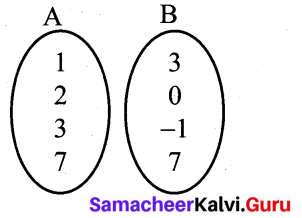It is not a relation there is no element as 1 in B.
(ii) R2 = {(-1, 1)}
It is not [∵ -1 ∉ A, 1 ∉ B]
(iii) R3 = {(2, -1), (7, 7), (1, 3)}
It is a relation.
R4 = {(7,-1), (0, 3), (3, 3), (0, 7)}
It is also not a relation. [∵ 0 ∉ A]

Ex 1.2 Class 10 Samacheer Question 2.
Let A = {1, 2, 3, 4, ….., 45} and R be the relation defined as 'is square of ' on A. Write R as a subset of A × A. Also, find the domain and range of R.
A = {1,2, 3, 4 . . . . 45}
The relation is defined as 'is square of’
R = {(1,1) (2, 4) (3, 9)
(4, 16) (5,25) (6, 36)}
Domain of R = {1, 2, 3, 4, 5, 6}
Range of R = {1, 4, 9, 16, 25, 36}

Exercise 1.2 Class 10 Maths Samacheer Question 3.
A Relation R is given by the set {(x, y) /y = x + 3, x ∈ {0, 1, 2, 3, 4, 5}}. Determine its domain and range.
Solution:
x = {0, 1, 2, 3, 4, 5}
y = x + 3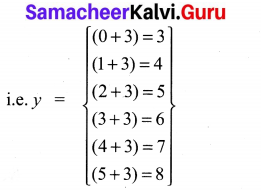⇒ y = {3, 4, 5, 6, 7, 8}
R = {(x, y)}
= {(0, 3),(1, 4),(2, 5),(3, 6), (4, 7), (5, 8)}
Domain of R = {0, 1, 2, 3, 4, 5}
Range of R = {3, 4, 5, 6, 7, 8}

10th Maths Exercise 1.2 Question 4.
Represent each of the given relation by (a) an arrow diagram, (b) a graph and (c) a set in roster form, wherever possible.
(i) {(x, y)|x = 2y,x ∈ {2, 3, 4, 5},y ∈ {1, 2, 3, 4)
(ii) {(x, y)|y = x + 3, x, y are natural numbers <10}
Solution:
(i){(x, y)|x = 2y, x ∈ {2, 3, 4, 5},y ∈ {1, 2, 3, 4}} R = (x = 2y)
2 = 2 × 1 = 2
4 = 2 × 2 = 4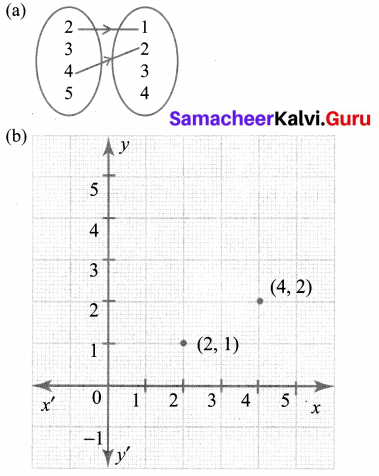(c) {(2, 1), (4, 2)}
(ii) {(x, y)|y = x + 3, x,+ are natural numbers <10}
x = {1, 2, 3, 4, 5, 6, 7, 8, 9} R = (y = x + 3)
y = {1, 2, 3, 4, 5, 6, 7, 8, 9}
R = {(1, 4), (2, 5), (3, 6), (4, 7), (5, 8), (6, 9)}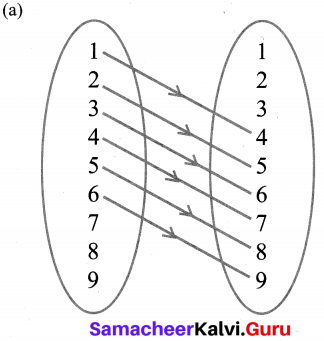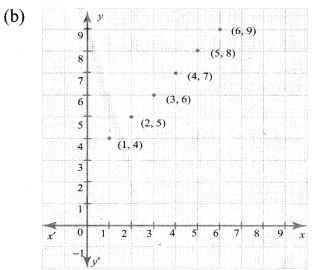(c) R = {(1, 4), (2, 5), (3, 6), (4, 7), (5, 8), (6, 9)}

10th Maths Exercise 1.2 Answers Question 5.
A company has four categories of employees given by Assistants (A), Clerks (C), Managers (M) and an Executive Officer (E). The company provide ₹10,000, ₹25,000, ₹50,000 and ₹1,00,000 as salaries to the people who work in the categories A, C, M and E respectively. If A1, A2, A3, A4 and As were Assistants; C1, C2, C3, C4 were Clerks; M1, M2, M3 were managers and E1,E2 were Executive officers and if the relation R is defined by xRy, where x is the salary given to person y, express the relation R through an ordered pair and an arrow diagram.
Solution:
A – Assistants → A1, A2, A3, A4, A5
C – Clerks → C1, C2, C3, C4
D – Managers → M1, M2, M3
E – Executive officer → E1, E2
(a) R = {(10,000, A1), (10,000, A2), (10,000, A3),
(10,000, A4), (10,000, A5), (25,000, C1),
(25,000, C2), (25,000, C3), (25,000, C4),
(50,000, M1), (50,000, M2), (50,000, M3),
(1,00,000, E1), (1,00,000, E2)}

(b)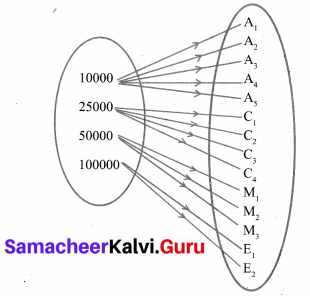## TN Class 10th Maths Solutions Chapter 1 Relations and Functions Ex 1.3

Question 1.
Let f = {(x, y)|x, y ∈ N and y = 2x} be a relation on N. Find the domain, co-domain and range. Is this relation a function?
Solution:
F = {(x, y)|x, y ∈ N and y = 2x}
x = {1, 2, 3,…}
y = {1 × 2, 2 × 2, 3 × 2, 4 × 2, 5 × 2 …}
R = {(1, 2), (2, 4), (3, 6), (4, 8), (5, 10),…}
Domain of R = {1, 2, 3, 4,…},
Co-domain = {1, 2, 3…..}
Range of R = {2, 4, 6, 8, 10,…}
Yes, this relation is a function.

Question 2.
Let X = {3, 4, 6, 8}. Determine whether the relation R = {(x, f(x))|x ∈ X, f(x) = x2 + 1} is a function from X to N ?
Solution:
x = {3,4, 6, 8}
R = ((x, f(x))|x ∈ X, f(x) = X2 + 1}
f(x) = x2 + 1
f(3) = 32 + 1 = 10
f(4) = 42 + 1 = 17
f(6) = 62 + 1 = 37
f(8) = 82 + 1 = 65R = {(3, 10), (4, 17), (6, 37), (8, 65)}
R = {(3, 10), (4, 17), (6, 37), (8, 65)}
Yes, R is a function from X to N.Question 3.
Given the function
f : x → x2 – 5x + 6, evaluate
(i) f(-1)
(ii) f(2 a)
(iii) f(2)
(iv) f(x – 1)
f(x) = x2 – 5x + 6
(i) f (-1) = (-1)2 – 5 (-1) + 6 = 1 + 5 + 6 = 12
(ii) f (2a) = (2a)2 – 5 (2a) + 6 = 4a2 – 10a + 6
(iii) f(2) = 22 – 5(2) + 6 = 4 – 10 + 6 = 0
(iv) f(x – 1) = (x – 1)2 – 5 (x – 1) + 6
= x2 – 2x + 1 – 5x + 5 + 6
= x2 – 7x + 12

Question 4.
A graph representing the function f(x) is given in figure it is clear that f(9) = 2.(i) Find the following values of the function
(a) f(0)
(b) f(7)
(c) f(2)
(d) f(10)
(ii) For what value of x is f (x) = 1?
(iii) Describe the following
(i) Domain
(ii) Range.
(iv) What is the image of 6 under f?
Solution:
From the graph
(a) f(0) = 9
(b) f(7) = 6
(c) f(2) = 6
(d) f(10) = 0
(ii) At x = 9.5, f(x) = 1
(iii) Domain = {0, 1, 2, 3, 4, 5, 6, 7, 8, 9, 10}
= {x |0 < x < 10, x ∈ R}
Range = {x|0 < x < 9, x ∈ R}
= {0, 1, 2, 3, 4, 5, 6, 7, 8, 9}
(iv) The image of 6 under f is 5.Question 5.
Let f(x) = 2x + 5. If x ≠ 0 then find f(x+2)−f(2)x
Solution:
Given f(x) = 2x + 5, x ≠ 0.Question 6.
A function fis defined by f(x) = 2x – 3
(i) find f(0)+f(1)2
(ii) find x such that f(x) = 0.
(iii) find x such that f(x) = x.
(iv) find x such that f(x) = f(1 – x).
Solution:
Given f(x) = 2x – 3
(i) find f(0)+f(1)2
f(0) = 2(0) – 3 = -3
f(1) = 2(1) – 3 = -1
∴ f(0)+f(1)2=−3−12=−42 = -2

(ii) f(x) = 0
⇒ 2x – 3 = 0
2x = 3
x = 32

(iii) f(x) = x
⇒ 2x – 3 = x ⇒ 2x – x = 3
x = 3

(iv) f(x) = f(1 – x)
2x – 3 = 2(1 – x) – 3
2x – 3 = 2x – 2x – 3
2x + 2x = 2 – 3 + 3
4x = 2
x = 24
x = 12

Question 7.
An open box is to be made from a square piece of material, 24 cm on a side, by cutting equal squares from the corners and turning up the sides as shown in figure. Express the volume V of the box as a function of x.Solution:
Volume of the box = Volume of the cuboid
= l × b × h cu. units
Here l = 24 – 2x
b = 24 – 2x
h = x
∴ V = (24 – 2x) (24 – 2x) × x
= (576 – 48x – 48x + 4x2)x
V = 4x3 – 96x2 + 576x

Question 8.
A function f is defined bv f(x) = 3 – 2x . Find x such that f(x2) = (f(x))2.
Solution:
f(x) = 3 – 2x
f(x2) = 3 – 2x2Question 9.
A plane is flying at a speed of 500 km per hour. Express the distance d travelled by the plane as function of time r in hours.
Speed of the plane = 500 km/hr
Distance travelled in 't' hours
= 500 × t (distance = speed × time)
= 500 t

Question 10.
The data in the adjacent table depicts the length of a woman’s forehand and her corresponding height. Based on this data, a student finds a relationship between the height (y) and the forehand length(x) as y = ax + b, where a, b are constants.(i) Check if this relation is a function.
(ii) Find a and b.
(iii) Find the height of a woman whose forehand length is 40 cm.
(iv) Find the length of forehand of a woman if her height is 53.3 inches.
Solution:
(i) Given y = ax + b …………. (1)
The ordered pairs are R = {(35, 56) (45, 65) (50, 69.5) (55, 74)}
∴ Hence this relation is a function.Substituting a = 0.9 in (2) we get
⇒ 65 = 45(.9) + b
⇒ 65 = 40.5 + b
⇒ b = 65 – 40.5
⇒ b = 24.5
∴ a = 0.9, b = 24.5
∴ y = 0.9x + 24.5
(iii) Given x = 40 , y = ?
∴ (4) → y = 0.9 (40) + 24.5
⇒ y = 36 + 24.5
⇒ y = 60.5 inches
(iv) Given y = 53.3 inches, x = ?
(4) → 53.3 = 0.9x + 24.5
⇒ 53.3 – 24.5 = 0.9x
⇒ 28.8 = 0.9x
⇒ x = 28.80.9 = 32 cm
∴ When y = 53.3 inches, x = 32 cm

Exercise 1.4 Class 10 Maths Samacheer Question 1.
Determine whether the graph given below represent functions. Give reason for your answers concerning each graph.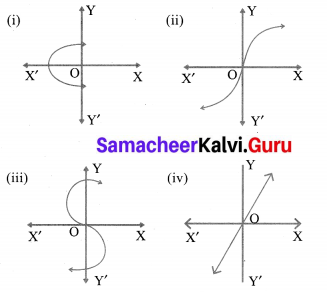Solution: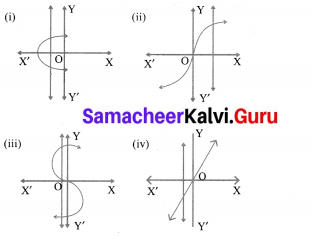(i) It is not a function. The graph meets the vertical line at more than one points.
(ii) It is a function as the curve meets the vertical line at only one point.
(iii) It is not a function as it meets the vertical line at more than one points.
(iv) It is a function as it meets the vertical line at only one point.

10th Maths Exercise 1.4 Samacheer Kalvi Question 2.
Let f :A → B be a function defined by f(x) = x2 – 1, Where A = {2, 4, 6, 10, 12},
B = {0, 1, 2, 4, 5, 9}. Represent f by
(i) set of ordered pairs;
(ii) a table;
(iii) an arrow diagram;
(iv) a graph
Solution:
f: A → B
A = {2, 4, 6, 10, 12}, B = {0, 1, 2, 4, 5, 9}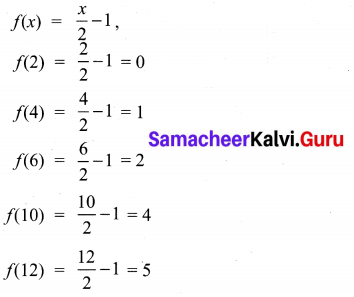(i) Set of ordered pairs
= {(2, 0), (4, 1), (6, 2), (10, 4), (12, 5)}
(ii) a table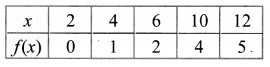(iii) an arrow diagram;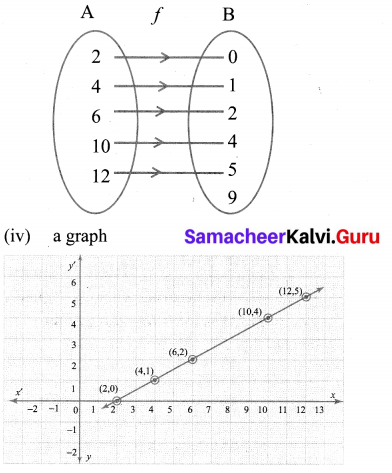Ex 1.4 Class 10 Samacheer Question 3.
Represent the function f = {(1, 2),(2, 2),(3, 2), (4,3), (5,4)} through
(i) an arrow diagram
(ii) a table form
(iii) a graph
Solution:
f = {(1, 2), (2, 2), (3, 2), (4, 3), (5, 4)}
(i) An arrow diagram.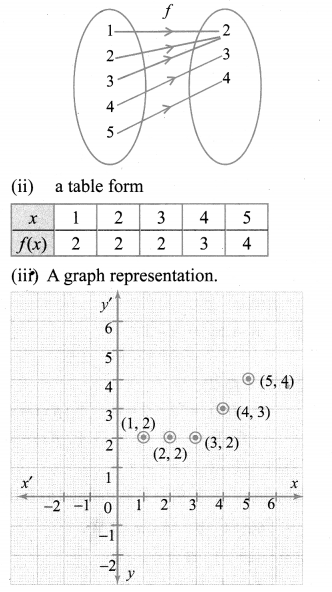10th Maths Exercise 1.4 Question 4.
Show that the function f : N → N defined by f{x) = 2x – 1 is one – one but not onto.
Solution:
f: N → N
f(x) = 2x – 1
N = {1, 2, 3, 4, 5,…}
f(1) = 2(1) – 1 = 1
f(2) = 2(2) – 1 = 3
f(3) = 2(3) – 1 = 5
f(4) = 2(4) – 1 = 7
f(5) = 2(5) – 1 = 9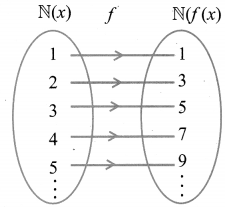In the figure, for different elements in x, there are different images in f(x).
Hence f : N → N is a one-one function.
A function f: N → N is said to be onto function if the range of f is equal to the co-domain of f
Range = {1, 3, 5, 7, 9,…}
Co-domain = {1, 2, 3,..}
But here the range is not equal to co-domain. Therefore it is one-one but not onto function.

10th Maths 1.4 Exercise Question 5.
Show that the function f: N → N defined by f (m) = m2 + m + 3 is one – one function.
Solution:
f: N → N
f(m) = m2 + m + 3
N = {1, 2, 3, 4, 5…..}m ∈ N
f{m) = m2 + m + 3
f(1) = 12 + 1 + 3 = 5
f(2) = 22 + 2 + 3 = 9
f(3) = 32 + 3 + 3 = 15
f(4) = 42 + 4 + 3 = 23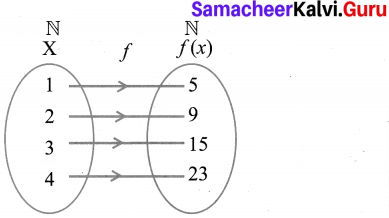In the figure, for different elements in the (X) domain, there are different images in f(x). Hence f: N → N is a one to one but not onto function as the range of f is not equal to co-domain.
Hence it is proved.

10th Maths Ex 1.4 Question 6.
Let A = {1,2, 3, 4} and B = N .
Let f: A → B be defined by f(x) = x3 then,
(i) find the range of f
(ii) identify the type of function
A = {1,2, 3,4}
B = {1,2, 3, 4, 5,….}
f(x) = x3
f(1) = 13 = 1
f(2) = 23 = 8
f(3) = 33 = 27
f(4) = 43 = 64
(i) Range = {1,8, 27, 64}
(ii) one -one and into function.

10th Maths Exercise 1.4 Answers Question 7.
In each of the following cases state whether the function is bijective or not. Justify your answer.
(i) f: R → R defined by f(x) = 2x + 1
(ii) f: R → R defined by f(x) = 3 – 4x2
Solution:
(i) f : R → R
f(x) = 2x + 1
f(1) = 2(1) + 1 = 3
f(2) = 2(2) + 1 = 5
f(-1) = 2(-1) + 1 = -1
f(0) = 2(0) + 1 = 1
It is a bijective function. Distinct elements of A have distinct images in B and every element in B has a pre-image in A.
(ii) f: R → R; f(x) = 3 – 4x2
f(1) = 3 – 4(12) = 3 – 4 = -1
f(2) = 3 – 4(22) = 3 – 16 = -13
f(-1) = 3 – 4(-1)2 = 3 – 4 = -1
It is not bijective function since it is not one-one

Samacheer Kalvi 10th Maths Book Graph Solution Question 8.
Let A = {-1, 1} and B = {0, 2}. If the function f: A → B defined by f(x) = ax + b is an onto function? Find a and b.
Solution:
A= {-1, 1},B = {0, 2}
f: A → B, f(x) = ax + b
f(-1) = a(-1) + b = -a + b
f(1) = a(1) + b = a + b
Since f(x) is onto, f(-1) = 0
⇒ -a + b = 0 …(1)
& f(1) = 2
⇒ a + b = 2 …(2)
-a + b = 0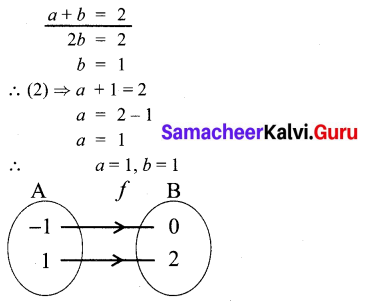10th Maths Exercise 1.4 In Tamil Question 9.
If the function f is defined by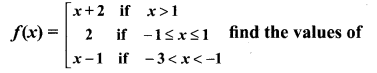(i) f(3)
(ii) f(0)
(iii) f(-1.5)
(iv) f(2) + f(-2)
Solution:
(i) f(3) ⇒ f(x) = x + 2 ⇒ 3 + 2 = 5
(ii) f(0) ⇒ 2
(iii) f (- 1.5) = x – 1
= -1.5 – 1 = -2.5
(iv) f(2) + f(-2)
f(2) = 2 + 2 = 4 [∵ f(x) = x + 2]
f(-2) = -2 – 1 = -3 [∵ f(x) = x – 1]
f(2) + f(-2) = 4 – 3 = 1

Samacheer Kalvi 10th Maths Exercise 1.4 Question 10.
A function f: [-5,9] → R is defined as follows: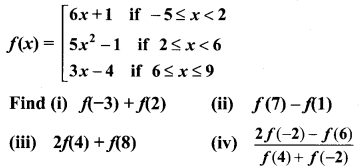Solution:
f : [-5, 9] → R
(i) f(-3) + f(2)
f(-3) = 6x + 1 = 6(-3) + 1 = -17
f(2) = 5 × 2 – 1 = 5(22) – 1 = 19
∴ f(-3) + f(2) = -17 + 19 = 2

(ii) f(7) – f(1)
f(7) = 3x – 4 = 3(7) – 4 = 17
f(1) = 6x + 1 = 6(1) + 1 = 7
f(7) – f(1) = 17 – 7 = 10

(iii) 2f(4) + f(8)
f(4) = 5x2 – 1 = 5 × 42 – 1 = 79
f(8) = 3x – 4 = 3 × 8 – 4 = 20
∴ 2f(4) + f(8) = 2 × 79 + 20 = 178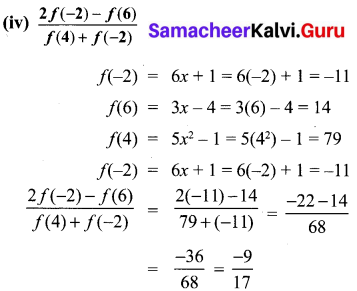10th Maths 1.4 Question 11
The distance S an object travels under the influence of gravity in time t seconds is 1 2 given by S(t) = 13gt2 + at + b, where, (g is the acceleration due to gravity), a, b are constants. Check if the function S(t) is one-one.
S(t) = 12gt2 + at + b
Let the time be 1, 2, 3 …. n seconds
S(1) = 12g(1)2 + a(1) + b
= g2 + a + b
S(2) = 12 g(2)2 + a(2) + b
= 4g2 + 2a + b
= 2g + 2a + b
S(3) = 12 g(3)2 + a(3) + 6
= 92 g + 3a + b
For every different value of t, there will be different distance.
∴ It is a one-one function.

Samacheer Kalvi 10th Maths Book Graph Solutions Question 12.
The function ‘t’ which maps temperature in Celsius (C) into temperature in Fahrenheit (F) is defined by t(C)= F where F = 95 C + 32 . Find,
(i) t(0)
(ii) t(28)
(iii) t(-10)
(iv) the value of C when t(C) = 212
(v) the temperature when the Celsius value is equal to the Farenheit value.
Solution: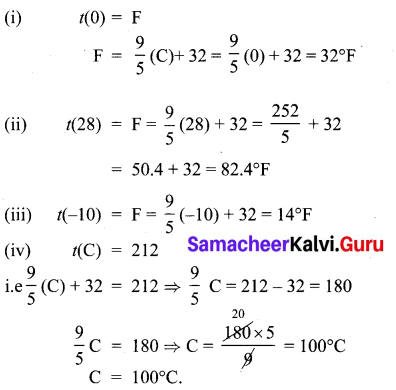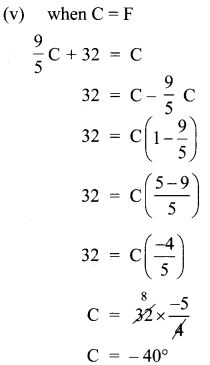f(x) = 2x – 1, g(x) = x+12, fog = gof = xQuestion 4.
(i) If f (x) = x2 – 1, g(x) = x – 2 find a, if g o f(a) = 1.
(a) Find k, if f(k) = 2k -1 and
fof (k) = 5.
(i) f(x) = x2 – 1 ; g(x) = x – 2 .
gof = g [f(x)]
= g(x2 – 1)
= x2 – 1 – 2
= x2 – 3
given gof (a) = 1
a2 – 3 = 1 [But go f(x) = x2 – 3]
a2 = 4
a = 4 = ± 2
The value of a = ± 2

(ii) f(k) = 2k – 1 ; fof(k) = 5
fof = f[f(k)]
= f(2k – 1)
= 2(2k – 1) – 1
= 4k – 2 – 1
= 4k – 3
fof (k) = 5
4k – 3 = 5
4k = 5 + 3
4k = 8
k = 84 = 2
The value of k = 2

Question 5.
Let A,B,C ⊂ N and a function f: A → B be defined by f(x) = 2x + 1 and g : B → C be defined by g(x) = x2. Find the range of fog and gof
Solution:
f(x) = 2x + 1
g(x) = x2
fog(x) = fg(x)) = f(x2) = 2x2 + 1
gof(x) = g(f(x)) = g(2x + 1) = (2x + 1)2
= 4x2 + 4x + 1
Range of fog is
{y/y = 2x2 + 1, x ∈ N}
Range of gof is
{y/y = (2x + 1)2, x ∈ N}.

Question 6.
Let f(x) = x2 – 1. Find (i) fof (ii) fofof
f(x) = x2 – 1
(i) fof = f[f{x)]
= f(x2 – 1)
= (x2 – 1)2 – 1
= x4 – 2x2 + 1 – 1
= x4 – 2x2

(ii) fofof = fof[f(x)]
= fof (x2 – 1)
= f(x2 – 1)2 – 1
= f(x4 – 2x2 + 1 – 1)
= f (x4 – 2x2)
fofof = (x4 – 2x2)2 – 1

Question 7.
If f: R → R and g : R → R are defined by f(x) = x5 and g(x) = x4 then check if f,g are one-one and fog is one-one?
Solution:
f(x) = x5
g(x) = x4
fog = fog(x) = f(g(x)) = f(x4)
= (x4)5 = x20
f is one-one, g is not one-one.
∵ g(1) = 14 = 1
g(-1) = ( -1)4 = 1
Different elements have same images
fog is not one-one. [∵ fog (1) = fog (-1) = 1]

Question 8.
Consider the functions f(x), g(x), h(x) as given below. Show that
(f o g) o h = f o(g o h) in each case.
(i) f(x) = x – 1, g(x) = 3x + 1 and h(x) = x2
(ii) f(x) = x2, g(x) = 2x and h(x) = x + 4
(iii) f(x) = x – 4, g(x) = x2 and h(x) = 3x – 5
(i) f(x) = x – 1, g (x) = 3x + 1, h(x) = x2
fog (x) = f[g(x)]
= f(3x + 1)
= 3x + 1 – 1
fog = 3x
(fog) o h(x) = fog [h(x)] ,
= fog (x2)
= 3(x2)
(fog) oh = 3x2 …..(1)
goh (x) = g[h(x)]
= g(x2)
= 3(x2) + 1
= 3x2 +1
fo(goh) x = f [goh(x)]
= f[3x2 + 1]
= 3x2 + 1 – 1
= 3x2 ….(2)
From (1) and (2) we get
(fog) oh = fo (goh)
Hence it is verified

(ii) f(x) = x2 ; g (x) = 2x and h(x) = x + 4
(fog) x = f[g(x)]
= f (2x)
= (2x)2
= 4x2
(fog) oh (x) = fog [h(x)]
= fog (x + 4)
= 4(x + 4)2
= 4[x2 + 8x + 16]
= 4x2 + 32x + 64 …. (1)
goh (x) = g[h(x)]
= g(x + 4)
= 2(x + 4)
= 2x + 8
fo(goh) x = fo [goh(x)]
= f[2x + 8]
= (2x + 8)2
= 4×2 + 32x + 64 …. (2)
From (1) and (2) we get
(fog) oh = fo(goh)

(iii) f(x) = x – 4 ; g (x) = x2; h(x) = 3x – 5
fog (x) = f[g(x)]
= f(x2)
= x2 – 4
(fog) oh (x) = fog [h(x)]
= fog (3x – 5)
= (3x – 5)2 – 4
= 9x2 – 30x + 25 – 4
= 9x2 – 30x + 21 ….(1)
goh (x) = g[h(x)]
= g(3x – 5)
= (3x – 5)2
= 9x2 + 25 – 30x
fo(goh)x = f[goh(x)]
= f[9x2 – 30x + 25]
= 9x2 – 30x + 25 – 4
= 9x2 – 30x + 21 ….(2)
From (1) and (2) we get
(fog) oh = fo(goh)

Question 9.
Let f ={(-1, 3),(0, -1),(2, -9)} be a linear function from Z into Z . Find f(x).
Solution:
f ={(-1, 3), (0, -1), 2, -9)
f(x) = (ax) + b ………… (1)
is the equation of all linear functions.
∴ f(-1) = 3
f(0) = -1
f(2) = -9
f(x) = ax + b
f(-1) = -a + b = 3 …………… (2)
f(0) = b = -1
-a – 1 = 3 [∵ substituting b = – 1 in (2)]
-a = 4
a = -4
The linear function is -4x – 1. [From (1)]

Question 10.
In electrical circuit theory, a circuit C(t) is called a linear circuit if it satisfies the superposition principle given by C(at1 + bt2) = aC(t1) + bC(t2), where a, b are constants. Show that the circuit C(t) = 31 is linear.
Given C(t) = 3t
C(at1) = 3at1 …. (1)
C(bt2) = 3 bt2 …. (2)
C(at1) + C(bt2) = 3at1 + 3bt2
C(at1 + bt2) = 3at1 + 3bt2
= Cat1 + Cbt2 [from (1) and (2)]
∴ C(at1 + bt2) = C(at1 + bt2)
Superposition principle is satisfied.
∴ C(t) = 3t is a linear function.

## Tamilnadu Samacheer Kalvi 10th Maths Solutions Chapter 1 Relations and Functions Ex 1.6

Question 1.
If n(A × B) = 6 and A = {1, 3} then n(B) is
(1) 1
(2) 2
(3) 3
(4) 6
(3) 3
Hint:
If n(A × B) = 6
A = {1, 1}, n(A) = 2
n(B) = 3

Question 2.
A = {a, b,p}, B = {2, 3}, C = {p, q, r, s)
then n[(A ∪ C) × B] is ………….
(1) 8
(2) 20
(3) 12
(4) 16
(3) 12
Hint: A ∪ C = [a, b, p] ∪ [p, q, r, s]
= [a, b, p, q, r, s]
n (A ∪ C) = 6
n(B) = 2
∴ n [(A ∪ C)] × B] = 6 × 2 = 12

Question 3.
If A = {1, 2}, B = {1, 2, 3, 4}, C = {5, 6} and D = {5, 6, 7, 8} then state which of the following statement is true.
(1) (A × C) ⊂ (B × D)
(2) (B × D) ⊂ (A × C)
(3) (A × B) ⊂ (A × D)
(4) (D × A) ⊂ (B × A)
(1) (A × C) ⊂ (B × D)]
Hint:
A = {1, 2}, B = {1, 2, 3, 4},
C = {5, 6}, D ={5, 6, 7, 8}
A × C ={(1,5), (1,6), (2, 5), (2, 6)}
B × D = {(1, 5),(1, 6),(1, 7),(1, 8),(2, 5),(2, 6), (2, 7), (2, 8), (3, 5), (3, 6), (3, 7), (3, 8)}
∴ (A × C) ⊂ B × D it is true

Question 4.
If there are 1024 relations from a set A = {1, 2, 3, 4, 5} to a set B, then the number of elements in B is ………………….
(1) 3
(2) 2
(3) 4
(4) 8
(2) 2
Hint: n(A) = 5
n(A × B) = 10
(consider 1024 as 10)
n(A) × n(B) = 10
5 × n(B) = 10
n(B) = 105 = 2
n(B) = 2

Question 5.
The range of the relation R = {(x, x2)|x is a prime number less than 13} is
(1) {2, 3, 5, 7}
(2) {2, 3, 5, 7, 11}
(3) {4, 9, 25, 49, 121}
(4) {1, 4, 9, 25, 49, 121}
(3) {4, 9, 25, 49, 121}]
Hint:
R = {(x, x2)/x is a prime number < 13}
The squares of 2, 3, 5, 7, 11 are
{4, 9, 25, 49, 121}

Question 6.
If the ordered pairs (a + 2,4) and (5, 2a + 6) are equal then (a, b) is ………
(1) (2, -2)
(2) (5, 1)
(3) (2, 3)
(4) (3, -2)
(4) (3, -2)
Hint: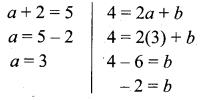The value of a = 3 and b = -2

Question 7.
Let n(A) = m and n(B) = n then the total number of non-empty relations that can be defined from A to B is
(1) mn
(2) nm
(3) 2mn – 1
(4) 2mn
(4) 2mn
Hint:
n(A) = m, n(B) = n
n(A × B) = 2mn

Question 8.
If {(a, 8),(6, b)} represents an identity function, then the value of a and 6 are respectively
(1) (8,6)
(2) (8,8)
(3) (6,8)
(4) (6,6)
(1) (8,6)
Hint: f = {{a, 8) (6, 6)}. In an identity function each one is the image of it self.
∴ a = 8, b = 6

Question 9.
Let A = {1, 2, 3, 4} and B = {4, 8, 9, 10}. A function f : A → B given by f = {(1, 4),(2, 8),(3, 9),(4, 10)} is a
(1) Many-one function
(2) Identity function
(3) One-to-one function
(4) Into function
(3) One-to one function
Hint:
A = {1, 2, 3, 4), B = {4, 8, 9,10}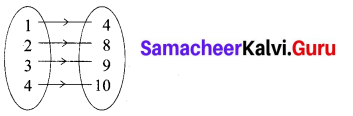Question 10.
If f(x) = 2x2 and g (x) = 13x, Then fog is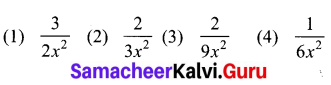(3) 29x2
Hint:
f(x) = 2x2
g(x) = 13x
fog = f(g(x)) = f(13x)=2(13x)2
= 2 × 19x2=29x2

Queston 11.
If f: A → B is a bijective function and if n(B) = 7 , then n(A) is equal to …………..
(1) 7
(2) 49
(3) 1
(4) 14
(1) 7
Hint:
n(B) = 7
Since it is a bijective function, the function is one – one and also it is onto.
n(A) = n(B)
∴ n(A) = 7

Question 12.
Let f and g be two functions given by f = {(0, 1), (2, 0), (3, -4), (4, 2), (5, 7)} g = {(0, 2), (1, 0), (2, 4), (-4, 2), (7, 0)} then the range of fog is
(1) {0, 2, 3, 4, 5}
(2) {-4, 1, 0, 2, 7}
(3) {1, 2, 3, 4, 5}
(4) {0, 1, 2}
(4) {0, 1, 2}
Hint:
gof = g(f(x))
fog = f(g(x))
= {(0, 2),(1, 0),(2, 4),(-4, 2),(7, 0)}
Range of fog = {0, 1, 2}

Question 13.
Let f (x) = 1+x2−−−−−√ then ………………..
(1) f(xy) = f(x) f(y)
(2) f(xy) > f(x).f(y)
(3) f(xy) < f(x). f(y)
(4) None of these
(3) f(xy) < f(x) . f(y)

Question 14.
If g = {(1, 1),(2, 3),(3, 5),(4, 7)} is a function given by g(x) = αx + β then the values of α and β are
(1) (-1, 2)
(2) (2, -1)
(3) (-1, -2)
(4) (1, 2)
(2) (2,-1)
Hint:
g(x) = αx + β
α = 2
β = -1
g(x) = 2x – 1
g(1) = 2(1) – 1 = 1
g(2) = 2(2) – 1 = 3
g(3) = 2(3) – 1 = 5
g(4) = 2(4) – 1 = 7

Question 15.
f(x) = (x + 1)3 – (x – 1)3 represents a function which is …………….
(1) linear
(2) cubic
(3) reciprocal
Hint: f(x) = (x + 1)3 – (x – 1)3
[using a3 – b3 = (a – b)3 + 3 ab (a – b)]
= (x + 1 – x + 1)3 + 3(x + 1) (x – 1)
(x + 1 – x + 1)
= 8 + 3 (x2 – 1)2
= 8 + 6 (x2 – 1)
= 8 + 6x2 – 6
= 6x2 + 2

## Tamilnadu Samacheer Kalvi 10th Maths Solutions Chapter 1 Relations and Functions Unit Exercise 1

10th Maths Unit Exercise 1 Question 1.
If the ordered pairs (x2 – 3x, y2 + 4y) and (-2, 5) are equal, then find x and y.
Solution:
(x2 – 3x, y2 + 4y) = (-2, 5)
x2 – 3x = -2
x2 – 3x + 2 = 0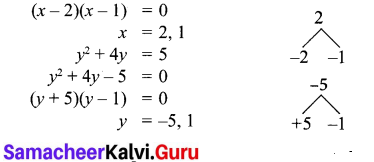10th Maths Chapter 1 Unit Exercise Question 2.
The cartesian product A × A has 9 elements among which (-1, 0) and (0,1) are found.
Find the set A and the remaining elements of A × A.
n(A × A) = 9
n(A) = 3
A = {-1,0,1}
A × A = {-1, 0, 1} × {-1, 0, 1}
A × A = {(-1,-1)(-1, 0) (-1, 1)
(0, -1) (0, 0) (0, 1)
(1,-1) (1, 0) (1, 1)}
The remaining elements of A × A =
{(-1, -1) (-1, 1) (0, -1) (0, 0) (1,-1) (1,0) (1,1)}

10th Maths Unit 1 Question 3.
Given that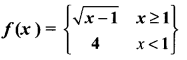(i) f(0)
(ii) f(3)
(iii) f(a + 1) in terms of a.(Given that a > 0)
Solution:
(i) f(0) = 4
(ii) f(3) = 31−−−−√=2–√
(iii) f(a + 1) = a+11−−−−−−−−√=a−−√

Samacheer Kalvi 10th Maths Book Solutions Question 4.
Let A = {9,10,11,12,13,14,15,16,17} and let f : A → N be defined by f(n) = the highest prime factor of n ∈ A. Write f as a set of ordered pairs and find the range of f.
A= {9, 10, 11, 12, 13, 14, 15, 16, 17}
f: A → N
f(x) = the highest prime factor n ∈ A
f = {(9, 3) (10, 5) (11, 11) (12, 3) (13, 13) (14, 7) (15, 5) (16, 2) (17, 17)}
Range of f = {3, 5, 11, 13, 7, 2, 17}
= {2, 3, 5, 7, 11, 13, 17}

10th Maths Exercise 1.1 Samacheer Kalvi Question 5.
Find the domain of the function f(x) = 1+11x2−−−−−√−−−−−−−−−−√−−−−−−−−−−−−−−−√
Solution:
f(x) = 1+11x2−−−−−√−−−−−−−−−−√−−−−−−−−−−−−−−−√
Domain of f(x) = {-1, 0, 1}
(x2 = 1, -1, 0, because 1x2−−−−−√ should be +ve, or 0)

10th Maths Unit Exercise Solutions Question 6.
If f (x) = x2, g(x) = 3x and h(x) = x – 2, Prove that (f o g)o h = f o(g o h).
f(x) = x2 ; g(x) = 3x and h(x) = x – 2
L.H.S. = (fog) oh
fog = f[g(x)]
= f(3x)
= (3x)2 = 9x2
(fog) oh = fog[h(x)]
= fog (x – 2)
= 9(x – 2)2
= 9[x2 – 4x + 4]
= 9x2 – 36x + 36 ….(1)
R.H.S. = fo(goh)
goh = g [h(x)]
= g(x – 2)
= 3(x – 2)
= 3x – 6
fo(goh) = fo [goh (x)]
= f(3x – 6)
= (3x – 6)2
= 9x2 – 36x + 36 ….(2)
From (1) and (2) we get
L.H.S. = R.H.S.
(fog) oh = fo {goh)

Samacheer Kalvi 10th Maths Exercise 1.1 Question 7.
A = {1, 2} and B = {1, 2, 3, 4} , C = {5, 6} and D = {5, 6, 7, 8} . Verify whether A × C is a subset of B × D?
Solution:
A = {1, 2), B = (1, 2, 3, 4)
C = {5, 6}, D = {5, 6, 7, 8)
A × C = {(1, 5), (1, 6), (2, 5), (2, 6)}
B × D = {(1, 5), (1, 6), (1, 7), (1, 8), (2, 5), (2, 6), (2, 7), (2, 8), (3, 5), (3, 6), (3, 7), (3, 8), (4, 5), (4, 6), (4, 7), (4, 8)}
(A × C) ⊂ (B × D) It is proved.

10th Maths Unit 1 Question Paper Question 8.
If f(x) = x1x+1, x ≠ 1 show that f(f(x)) = 1x, Provided x ≠ 0.
Solution: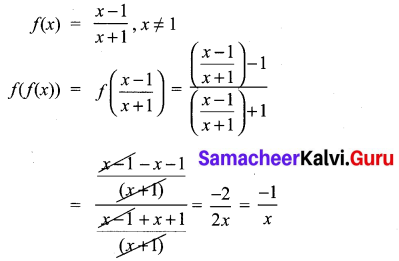Hence it is proved.

10th Maths Unit Exercise Question 9.
The function/and g are defined by f(x) = 6x + 8; g(x) = x23.
(i) Calculate the value of gg(12)
(ii) Write an expression for g f(x) in its simplest form.
Solution: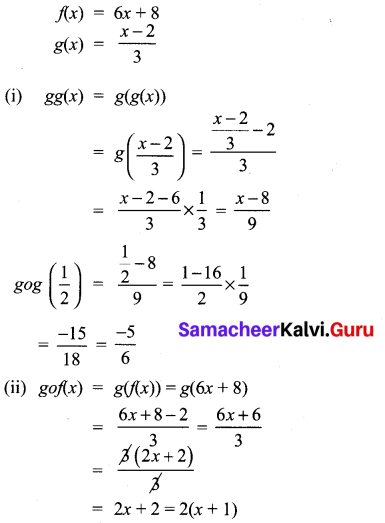10th Maths Chapter 1 Samacheer Kalvi Question 10.
Write the domain of the following real functions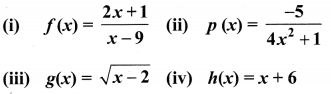Solution:
(i) f(x) = 2x+1x9
The denominator should not be zero as the function is a real function.
∴ The domain = R – {9}
(ii) p(x) = 54x2+1
The domain is R.
(iii) g(x) = x2−−−−−√
The domain = [2, ∝)
(iv) h(x) = x + 6
The domain is R.

## Tamilnadu Samacheer Kalvi 10th Maths Solutions Chapter 1 Relations and Functions Additional Questions

Question 1.
Let A = {1, 2, 3, 4} and B = {-1, 2, 3, 4, 5, 6, 7, 8, 9, 10, 11, 12} Let R = {(1, 3), (2, 6), (3,10), (4, 9)} ⊂ A × B be a relation. Show that R is a function and find its domain, co-domain and the range of R.
Domain of R = {1, 2, 3, 4}
Co-domain of R = B = {-1, 2, 3, 4, 5, 6, 7, 9, 10, 11, 12}
Range of R= {3, 6, 10, 9}

Question 2.
Let A = {0, 1, 2, 3} and B = {1, 3, 5, 7, 9} be two sets. Let f: A → B be a function given by f(x) = 2x + 1. Represent this function as (i) a set of ordered pairs (ii) a table (iii) an arrow and (iv) a graph.
Solution:
A = {0, 1, 2, 3}, B = {1, 3, 5, 7, 9}
f(x) = 2x + 1
f(0) = 2(0) + 1 = 1
f(1) = 2(1) + 1 = 3
f(2) = 2(2) + 1 = 5
f(3) = 2(3) + 1 = 7
(i) A set of ordered pairs.
f = {(0, 1), (1, 3), (2, 5), (3, 7)}
(ii) A table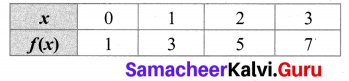(iii) An arrow diagram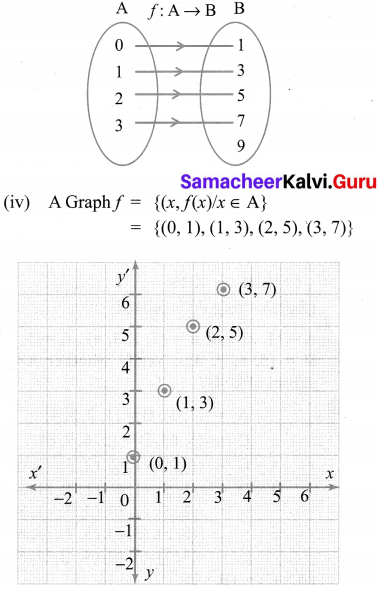Question 3.
State whether the graph represent a function. Use vertical line test.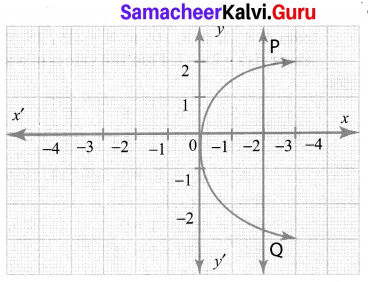Solution:
It is not a function as the vertical line PQ cuts the graph at two points.

Question 4.
Let f = {(2, 7), (3, 4), (7, 9), (-1, 6), (0, 2), (5, 3)} be a function from A = {-1, 0, 2, 3, 5, 7} to B = {2, 3, 4, 6, 7, 9}. Is this (i) an one-one function (ii) an onto function, (iii) both one- one and onto function?
Solution:
It is both one-one and onto function.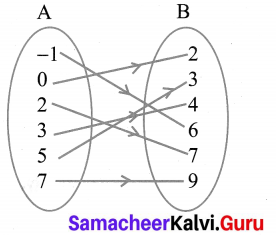All the elements in A have their separate images in B. All the elements in B have their preimage in A. Therefore it is one-one and onto function.

Question 5.
A function f: (-7,6) → R is defined as follows.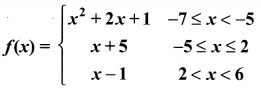Find (i) 2f(-4) + 3 f(2)
(ii) f(-7) – f(-3)
Solution: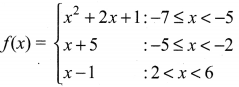(i) 2f(-4) + 3f(2)
f(-4) = x + 5 = -4 + 5 = 1
2f(-4) = 2 × 1 = 2
f(2) = x + 5 = 2 + 5 = 7
3f(2) = 3(7) = 21
∴ 2f(-4) + 3f(2) = 2 + 21 = 23

(ii) f(-7) = x2 + 2x + 1
= (-7)2 + 2(-7) + 1
= 49 – 14 + 1 = 36
f(3) = x + 5 = -3 + 5 = 2
f(-7) – f(-3) = 36 – 2 = 34

Question 6.
If A = {2,3, 5} and B = {1, 4} then find
(i) A × B
(ii) B × A
A = {2, 3, 5}
B = {1, 4}

(i) A × B = {2,3,5} × {1,4}
= {(2, 1) (2, 4) (3, 1) (3, 4) (5,1) (5, 4)}.

(ii) B × A = {1,4} × {2,3,5}
= {(1,2) (1,3) (1,5) (4, 2) (4, 3) (4, 5)}

Question 7.
Let A = {5, 6, 7, 8};
B = {- 11, 4, 7, -10, -7, – 9, -13} and
f = {(x,y): y = 3 – 2x, x ∈ A, y ∈ B}.
(i) Write down the elements of f.
(ii) What is the co-domain?
(iii) What is the range?
(iv) Identify the type of function.
Given, A = {5, 6, 7, 8},
B = {- 11,4, 7,-10,-7,-9,-13}
y = 3 – 2x
ie; f(x) = 3 – 2x
f(5) = 3 – 2 (5) = 3 – 10 = – 7
f(6) = 3 – 2 (6) = 3 – 12 = – 9
f(7) = 3 – 2(7) = 3 – 14 = – 11
f(8) = 3 – 2 (8) = 3 – 16 = – 13
(i) f = {(5, – 7), (6, – 9), (7, – 11), (8, – 13)}
(ii) Co-domain (B)
= {-11,4, 7,-10,-7,-9,-13} i
(iii) Range = {-7, – 9, -11,-13}
(iv) It is one-one function.

Question 8.
A function f: [1, 6] → R is defined as follows: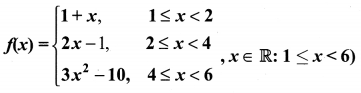Find the value of (i) f(5)
(ii) f(3)
(iii) f(2) – f(4).
Solution: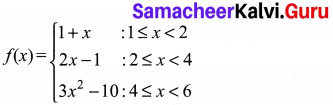(i) f(5) = 3x2 – 10
= 3 (52) – 10 = 75 – 10 = 65
(ii) f(3) = 2x – 1
= 2(3) – 1 = 6 – 1 = 5
(ii) f(2) – f(4)
f(2) = 2x – 1
= 2(2) – 1 = 3
f(4) = 3x2 – 10
= 3(42) – 10 = 38
∴ f(2) – f(4) = 3 – 38 = 35

Question 9.
The following table represents a function from A = {5, 6, 8, 10} to B = {19, 15, 9, 11}, where f(x) = 2x – 1. Find the values of a and b.
Solution: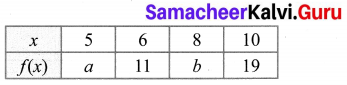A = {5, 6, 8, 10}, B = {19, 15, 9, 11}
f(x) = 2x – 1
f(5) = 2(5) – 1 = 9
f(8) = 2(8) – 1 = 15
∴ a = 9, b = 15

Question 10.
If R = {(a, -2), (-5, 6), (8, c), (d, -1)} represents the identity function, find the values of a,b,c and d.
Solution:
R = {(a, -2), (-5, b), (8, c), (d, -1)} represents the identity function.
a = -2, b = -5, c = 8, d = -1.

## How to Prepare using Samacheer Kalvi 10th Maths Chapter 1 Relations and Functions Notes PDF?

Students must prepare for the upcoming exams from Samacheer Kalvi 10th Maths Chapter 1 Relations and Functions Notes PDF by following certain essential steps which are provided below.

• Use Samacheer Kalvi 10th Maths Chapter 1 Relations and Functions notes by paying attention to facts and ideas.
• Pay attention to the important topics
• Refer TN Board books as well as the books recommended.
• Correctly follow the notes to reduce the number of questions being answered in the exam incorrectly
• Highlight and explain the concepts in details.

## Frequently Asked Questions on Samacheer Kalvi 10th Maths Chapter 1 Relations and Functions Notes

#### How to use Samacheer Kalvi 10th Maths Chapter 1 Relations and Functions Notes for preparation??

Read TN Board thoroughly, make separate notes for points you forget, formulae, reactions, diagrams. Highlight important points in the book itself and make use of the space provided in the margin to jot down other important points on the same topic from different sources.

#### How to make notes for Samacheer Kalvi 10th Maths Chapter 1 Relations and Functions exam?

Read from hand-made notes prepared after understanding concepts, refrain from replicating from the textbook. Use highlighters for important points. Revise from these notes regularly and formulate your own tricks, shortcuts and mnemonics, mappings etc.
Share: